# JP3969306B2 - Graph display control device and program - Google Patents

## Info

Publication number
JP3969306B2
JP3969306B2 JP2002377132A JP2002377132A JP3969306B2 JP 3969306 B2 JP3969306 B2 JP 3969306B2 JP 2002377132 A JP2002377132 A JP 2002377132A JP 2002377132 A JP2002377132 A JP 2002377132A JP 3969306 B2 JP3969306 B2 JP 3969306B2
Authority
JP
Japan
Prior art keywords
graph
display
displayed
parameter
operation
Prior art date
Legal status (The legal status is an assumption and is not a legal conclusion. Google has not performed a legal analysis and makes no representation as to the accuracy of the status listed.)
Active
Application number
JP2002377132A
Other languages
Japanese (ja)
Other versions
JP2004206577A (en
Inventor

Original Assignee
カシオ計算機株式会社
Priority date (The priority date is an assumption and is not a legal conclusion. Google has not performed a legal analysis and makes no representation as to the accuracy of the date listed.)
Filing date
Publication date
Application filed by カシオ計算機株式会社 filed Critical カシオ計算機株式会社
Priority to JP2002377132A priority Critical patent/JP3969306B2/en
Publication of JP2004206577A publication Critical patent/JP2004206577A/en
Application granted granted Critical
Publication of JP3969306B2 publication Critical patent/JP3969306B2/en
Application status is Active legal-status Critical
Anticipated expiration legal-status Critical

## Images

••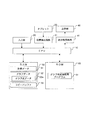•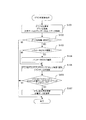•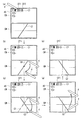•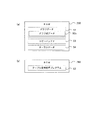•••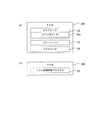•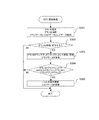••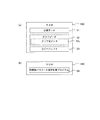•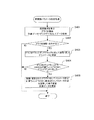•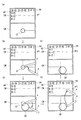•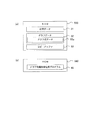•••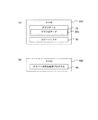•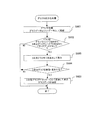•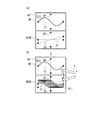•••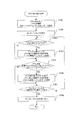•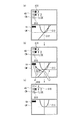••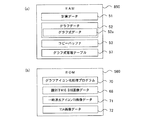•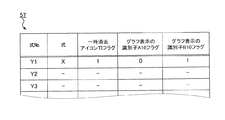•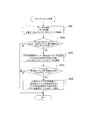••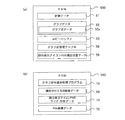•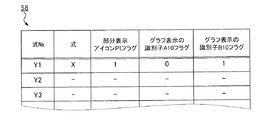•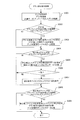•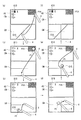## Description


BACKGROUND OF THE INVENTION
The present invention relates to a graph display control device and a program.

[Prior art]
2. Description of the Related Art Conventionally, an apparatus having a drawing function for drawing a geometric figure is known. For example, a scientific calculator (hereinafter referred to as “functional calculator”) having abundant functions such as a calculation function such as equation calculation, matrix calculation, and complex number calculation, a financial calculation function, and a statistical function is known. For example, the scientific calculator can display calculation results of various technical calculations using a calculation function in a graph and draw a figure of an inputted formula. Therefore, it is widely used for studying the relationship between character data such as mathematical formulas and graphs and figures in educational settings.

In addition, in the scientific calculator as described above, the outline of the graph to be displayed is input by handwriting with an input pen or the like, and the coordinates on the graph are input, thereby specifying the graph expression, and the graph expression There is known one having a function of displaying a graph based on (see, for example, Patent Document 1).

[Patent Document 1]
JP-A-9-282476

[Problems to be solved by the invention]
However, with a conventional scientific calculator, it is necessary to perform a series of processes according to the processing contents in order to draw a graph of an input function expression or figure expression or to display a graph expression or figure from a graph display. was there. For this reason, the user needs to understand the relationship between the operation contents of the scientific calculator and the processing executed by the scientific calculator according to the operation. That is, there is a problem that it is difficult for a user unfamiliar with the operation of the scientific calculator to make full use of the function of the scientific calculator.

Therefore, an object of the present invention is to improve the operability by simplifying the operation input and making it easy to understand the processing corresponding to the operation input in the graph display control device.

[Means for Solving the Problems]
In order to solve the above-mentioned problem, the graph display control device according to claim 1,
Formula storage means (for example, RAM 50 shown in FIG. 2; graph formula data 52a) that stores a graph formula including a parameter portion (eg, parameter portion CF1 shown in FIG. 4);
A graph drawing control means (for example, the CPU 10 shown in FIG. 2; step S101 shown in FIG. 3) for controlling the drawing of the graph-type graph stored in the formula storage means;
Graph deformation means (for example, CPU 10 shown in FIG. 2; step S106 shown in FIG. 3) for deforming the graph controlled by the graph drawing control means by a pointer operation;
When the graph is deformed by the graph deforming means, the parameter part value changing means (for example, shown in FIG. 2) updates the value of the parameter part of the graph formula stored in the formula storing means according to the deformed graph. CPU 10; step S107) shown in FIG.
With,
The graph formula stored in the formula storage means includes a plurality of parameter parts,
It further comprises parameter part selection means (for example, CPU 10 shown in FIG. 2; step S104 shown in FIG. 3) for selecting a parameter part from among the plurality of parameter parts.
The graph transformation means transforms the graph controlled by the graph drawing control means so as to vary the parameter part selected by the parameter part selection means (for example, the CPU 10 shown in FIG. 2; FIG. 3 Step S105),
The parameter part value changing means is means for changing the value of the parameter part selected by the parameter part selecting means according to the graph deformed by the graph deforming means (for example, the CPU 10 shown in FIG. 2; FIG. 3 Step S107) shown
It is characterized by that.

Here, the “parameter portion” refers to a constant or a coefficient constituting a calculation formula (graph formula) regardless of whether it is known or unknown. For example, if “y = 2x + b”, “2” and “b” are parameter parts, “x” and “y” as variables, and “=” and “+” as operation symbols. The part excluding is the parameter part.

Claim 1InAccording to the described invention, a graph expression including a parameter portion is stored, and after drawing the graph of this graph expression, the graph can be deformed by a pointer operation, and when the graph is deformed, the deformed graph The control of updating the value of the parameter portion can be performed according to the above. That is, the graph can be deformed by a pointer operation, and the graph formula can be updated according to the deformation of the graph. Therefore, it is possible to simplify the operation input, easily understand the process corresponding to the operation input, and improve the operability.Furthermore, when the graph is deformed by selecting and changing any of the plurality of parameter parts included in the graph expression, it is possible to perform control to change the value of the selected parameter part.

Claims2As in the invention described in claim 1,InIn the described graph display control device,
Expression display for performing control to update and display the graph expression stored in the expression storage means according to the value of the parameter part changed by the parameter part value changing means when the graph is deformed by the graph changing means You may comprise so that a control means may be further provided.

Claim2According to the invention described in the above, control is performed to display the graph expression. However, when the graph is deformed, the value of the parameter part of the graph expression is changed, and the graph expression that is being displayed is controlled to be updated. It can be performed.

DETAILED DESCRIPTION OF THE INVENTION
Embodiments of the present invention will be described below with reference to the drawings. In the following, the case where the present invention is applied to a graph scientific calculator which is a kind of electronic apparatus (graph display control device) having a graph display function will be described, but the present invention is not limited to this. Absent.

FIG. 1 is a diagram showing an example of the appearance of a graph scientific calculator (hereinafter simply referred to as “functional calculator”) 1 to which the present invention is applied. According to the figure, the scientific calculator 1 includes a display 2, a key group 3, and an input pen 4. Each key constituting the key group 3 is assigned a unique function, and the user operates the scientific calculator 1 by pressing these keys. Further, a tablet (touch panel) 30 to be described later is integrally provided on the display 2, and the user can also operate the scientific calculator 1 by a touch operation on the display 2 using the input pen 4. .

The scientific calculator 1 also includes, for example, a calculation application program (hereinafter referred to as “calculation application” as appropriate) for realizing a calculation function for calculating formulas, tables, lists, and the like, and a graph for realizing a graph drawing function. An application program (hereinafter referred to as “graph application” as appropriate) is installed.

Hereinafter, first to ninth embodiments of the scientific calculator 1 will be described with reference to the drawings.

Here, as a premise of each embodiment, the copy operation is to specify desired data displayed on the screen of the display 2 with a pointing device such as an input pen 4 or a mouse (pointer operation) and execute a copy command. It is an operation to do. The paste operation is an operation for specifying a data paste position (pointer operation) using a pointing device or the like and executing a paste command after the copy operation.

In the following embodiments, the copy operation and paste operation are realized by a drag and drop operation using the input pen 4. The drag operation is an operation of sliding the input pen 4 on the screen of the display 2. The drop operation is an operation of releasing the input pen 4 that is in contact with the screen after the drag operation from the screen. The drag and drop operation refers to this series of operations.

That is, the copy operation is realized by specifying desired data on the screen using the input pen 4 and then dragging the specified data. The paste operation is realized by moving the dragged data onto a desired screen by a drop operation. This drop position corresponds to the pasting position designated by the paste operation.

Note that the copy operation and the paste operation may be realized by other operations instead of the drag-and-drop operation. For example, the copy menu may be selected on the copy source screen, and the paste menu may be selected on the copy destination screen.

1. First embodiment
In the first embodiment, a parameter part included in an expression displayed on an expression display screen (hereinafter referred to as “calculation window CW”) of a calculation application is selected, and the value of the parameter part can be changed. . Then, after the graph displayed on the graph display screen of the graph application (hereinafter referred to as “graph window GW”) is moved or deformed according to the operation of the input pen 4, the following processing is performed. That is, when a copy operation (copy operation) is performed on the graph window GW and then a paste operation (paste operation) is performed on the calculation window CW, the graph window GW displays the calculation window CW based on the data specified by the copy operation. The control of updating the displayed expression is performed.

1.1 Internal configuration
FIG. 2 is a block diagram illustrating an example of an internal configuration of the scientific calculator 1 according to the first embodiment. According to the figure, the scientific calculator 1 includes a CPU 10, an input unit 20, a tablet 30, a position detection circuit 31, a display unit 40, a display drive circuit 41, a RAM 50, and a ROM 60.

The CPU 10 executes processing based on a predetermined program in accordance with an input instruction, performs an instruction to each function unit, data transfer, and the like, and controls the scientific calculator 1 in an integrated manner. Specifically, the CPU 10 reads a program stored in the ROM 60 in response to an operation signal input from the input unit 20 or the tablet 30, and executes processing according to the program. Then, the processing result is stored in the RAM 50, and a display signal for displaying the processing result is appropriately output to the display drive circuit 41 to display the corresponding display information on the display unit 40.

The input unit 20 is an input device provided with keys necessary for input of numerical values and mathematical formulas, function selection, and the like, and outputs a pressed signal of a pressed key to the CPU 10. By key input in the input unit 20, for example, instructions for starting various applications, execution of graphic drawing processing, input of mathematical expressions, execution of arithmetic processing, termination of processing and cancellation of modes, pointer operations, various selection operations, and the selection operations An input means such as a confirmation instruction is realized. The input unit 20 corresponds to the key group 3 shown in FIG.

The scientific calculator 1 includes a tablet 30 that is a touch panel as an input device. The tablet 30 is an input device in which a device such as an instruction pen (corresponding to the input pen 4 shown in FIG. 1) that indicates a position on the display unit 40 and a device that detects the position of the specified display unit 40 are combined. It is. A position detection circuit 31 connected to the tablet 30 detects position coordinates designated by the tablet 30. If this tablet 30 is used, the position in the display part 40 can be specified finely, and the input means in the input part 20 mentioned above by the touch operation of the display part 40 using the tablet 30 is realizable.

The display drive circuit 41 controls the display unit 40 based on a display signal input from the CPU 10 to display various screens. The display unit 40 includes an LCD (Liquid Crystal Display), an ELD (Electro Luminescent Display), or the like, and displays, for example, a display screen shown in FIG. The display unit 40 corresponds to the display 2 in FIG. 1 and is formed integrally with the tablet 30.

The RAM 50 includes a storage area (memory) that temporarily stores various processing programs executed by the CPU 10 and data related to the execution of these programs. In particular, in the present embodiment, a storage area that holds calculation data 51 that is data related to a calculation formula displayed in the calculation window CW, graph data 52 that is dot data of a graph drawn in the graph window GW, and a copy operation Is provided with a copy buffer 53 for holding the data designated by. The graph data 52 includes graph formula data (graph formula data) 52a for drawing a graph.

The ROM 60 stores various initial settings, hardware inspections, and initial programs for loading necessary programs. The CPU 10 sets the operating environment of the scientific calculator 1 by executing this initial program when the scientific calculator 1 is powered on.

The ROM 60 also implements various application programs such as a calculation application and a graph application, various processing programs related to the operation of the scientific calculator 1 such as various setting processes and various arithmetic processes, and various functions provided in the scientific calculator 1. Stores programs and data. In particular, in the present embodiment, a graph type conversion processing program 61 is stored.

The graph type conversion processing program 61 is a program for realizing the graph type conversion processing (see FIG. 3). When the CPU 10 executes the graph type conversion processing program 61, the graph type conversion processing is realized.

1.2 Operation
Next, the operation of the scientific calculator 1 in the first embodiment will be described.

FIG. 3 is a flowchart for explaining the operation of the scientific calculator 1 related to the graph type conversion processing, and FIG. 4 shows a transition example of the display screen displayed on the display unit 40 in the operation shown in the flowchart of FIG. FIG.

In the graph formula conversion process shown in FIG. 3, first, the CPU 10 starts execution of the calculation application to display the calculation window CW on the display unit 40, and starts execution of the graph application to display the graph window GW on the display unit 40. Display. Then, for example, the user inputs a graph expression at the cursor position of the calculation window CW using the key group 3 and designates a graph drawing command. Then, the CPU 10 displays the input graph formula data in the calculation window CW and stores it in the calculation data 51, draws a graph based on the calculation formula in the graph window GW, and draws the drawn graph and the graph formula data. Is stored in the graph data 52 (step S101).

FIG. 4A is a diagram showing an example of the display screen at this stage. According to the figure, a calculation window CW and a graph window GW are displayed on the display unit 40. In the calculation window CW, the graph expression C1 “Y1 = 2x + 3” is displayed. The parameter portion CF1 (“2” in the graph expression C1) and the parameter portion CF2 (“3” in the graph expression C1) in the graph expression C1 are displayed. ) Is blinking. In the graph window GW, a graph G1 based on the graph formula C1 is displayed.

When the CPU 10 detects that a command for moving or transforming the graph displayed in the graph window GW is input (step S102; YES), the CPU 10 determines whether or not there are a plurality of parameter portions included in the graph expression. (Step S103). If it is determined that there are a plurality of parameter parts (step S103; YES), the parameter part touched by the user using the input pen 4 is selected (step S104), and the process proceeds to step S105. On the other hand, when there is one parameter part included in the graph expression (step S103; NO), the CPU 10 proceeds to step S105 as it is.

FIG. 4B is a diagram illustrating an example of the display screen in step S104. According to the figure, the parameter portion CF1 (“2”) of the graph formula C1 displayed in the calculation window CW is selected and blinked, and the unselected parameter portion CF2 blinks after the original value “3” is confirmed. It is displayed without being. The user moves or deforms the graph G1 by dragging and dropping the graph G1 displayed on the graph window GW with the input pen 4. Here, since the parameter portion CF2 is fixed, the user can rotate the y-intercept “+3” of the graph G1 with the input pen 4 as the center point. A graph G2 after the rotation of the graph G1 is shown in FIG.

Next, the CPU 10 moves or transforms the graph in accordance with the drag and drop operation, and then updates the graph data 52 (step S105). Thereafter, the CPU 10 determines whether or not the graph is dragged and dropped from the graph window GW to the calculation window CW by an operation using the input pen 4 as shown in FIG. 4D (step S106). When it is determined that a drag and drop operation has been performed (step S106; YES), the CPU 10 calculates the graph expression of the graph after deformation or movement and displays it in the calculation window CW as shown in FIG. In addition to updating the existing graph formula to the graph formula, the calculation data 51 is updated (step S107).
When the processing described above is performed, the CPU 10 ends the graph type conversion processing.

As described above, according to the first embodiment, the user selects a parameter portion included in the graph expression displayed in the calculation window CW using the input pen 4, and then displays it in the graph window GW. The displayed graph can be transformed by a pointer operation using the input pen 4. When the deformed graph is moved to the calculation window CW by a drag-and-drop operation, the graph formula displayed in the calculation window CW is automatically updated.

Therefore, the graph can be changed and the graph expression can be changed with a simple operation, and the operability can be improved. In particular, since the graph by the input pen 4 is displayed and the graph expression is changed according to the deformed graph, it is easy to intuitively understand what processing is executed by the operation input. Yes, even users unfamiliar with scientific calculators can easily operate.

2. Second embodiment
Hereinafter, the second embodiment will be described in detail.
In the second embodiment, a table is displayed on a table display screen (hereinafter referred to as “table window TW”) of a calculation application, and a graph based on this table is displayed in a graph window GW. Is moved or deformed according to the operation of the input pen 4. Then, after a copy operation (copy operation) is performed on the graph window GW, when a paste operation (paste operation) is performed on the table window TW, the data is displayed on the table window TW based on the data specified by the copy operation. Control is performed to update the displayed table data.

2.1 Internal configuration
The configuration of the scientific calculator 1 in the second embodiment has a configuration in which the RAM 50 of the scientific calculator 1 shown in FIG. 2 in the first embodiment is replaced with the RAM 250, and the ROM 60 is replaced with the ROM 260. For this reason, the same code | symbol is attached | subjected to another identical function part, and the overlapping description is abbreviate | omitted.

FIG. 5A is a diagram illustrating a configuration of the RAM 250 according to the second embodiment. According to the figure, in the RAM 250, there are graph data 52 that is dot data displayed in the graph window GW, a copy buffer 53 that holds data designated by the copy operation, and data relating to the table displayed in the table window TW. Some table data 54 is stored. The graph data 52 includes graph formula data 52a for drawing a graph.

FIG. 5B is a diagram showing a configuration of the ROM 260 in the second embodiment. According to the figure, the ROM 260 stores a table conversion processing program 62.

The table conversion processing program 62 is a program for realizing table conversion processing (see FIG. 6). When the CPU 10 executes the table conversion processing program 62, the table conversion processing is realized.

2.2 Operation
Next, the operation of the scientific calculator 1 in the second embodiment will be described.

FIG. 6 is a flowchart for explaining the operation of the scientific calculator 1 according to the table conversion process, and FIG. 7 is a diagram showing a transition example of the display screen displayed on the display unit 40 in the operation shown in the flowchart of FIG. It is.

In the table conversion processing shown in FIG. 6, first, the CPU 10 starts execution of the calculation application to display the table window TW on the display unit 40, and starts execution of the graph application to display the graph window GW on the display unit 40. Let Then, the user designates a graph drawing command, for example, and inputs a desired numerical value at the cursor position of the table window TW using the key group 3. Then, the CPU 10 displays the input portion as table data in the table window TW and stores it in the table data 54, and draws a graph based on this table in the graph window GW. Is stored in the graph data 52 (step S201).

FIG. 7A is a diagram showing an example of a display screen at this stage. According to the figure, a table window TW and a graph window GW are displayed on the display unit 40. A table T1 including parameter parts TX1 (1, 2, 3, 4) and TY1 (1, 2, 3, 4) is displayed in the table window TW, and a graph G3 based on the table T1 is displayed in the graph window GW. Is displayed.

When the CPU 10 detects that a command for moving or deforming the graph has been input (step S202; YES), the CPU 10 enters a state in which the graph can be moved or deformed. Then, in accordance with the drag and drop operation using the input pen 4 by the user, the displayed graph is moved or deformed, and the graph data 52 is updated (step S203).

FIGS. 7B and 7C are diagrams showing examples of display screens in steps S202 and S203. According to FIG. 7B, the user uses the input pen 4 in the graph window GW to fix (1) the origin by pointing to the origin, and (2) displays the graph G3 on the input pen 4. The graph G3 is rotated around the origin by dragging and dropping with. As shown in FIG. 7C, the CPU 10 moves or deforms the graph G3 according to the drag-and-drop operation to obtain a graph G4.

Next, as shown in FIG. 7D, when the CPU 10 detects that the graph G4 after the movement or deformation has been dragged and dropped from the graph window GW to the table window TW (step S204; YES), the movement or deformation is performed. The table T2 corresponding to the later graph G4 is calculated, and the table T1 displayed in the calculation window CW is updated to the table T2 as shown in FIG. 7E, and the table data 54 is updated (step S205). . According to the figure, the value of the parameter portion TX1 (1, 2, 3, 4) is not changed in the table T2 compared to the original table T1, but the parameter portion TY1 (1, 2, 3, 4) is changed. The value of 4) changes to TY2 (-2, -4, -6, -8).
When the process described above is performed, the CPU 10 ends the table conversion process.

As described above, according to the second embodiment, the user can move or deform the graph displayed in the graph window GW by a pointer operation using the input pen 4. When the deformed graph is moved to the table window TW by a drag and drop operation, the table displayed in the table window TW is automatically updated.

Therefore, the graph can be deformed with a simple operation by a pointer operation such as a drag-and-drop operation, and the operability can be improved. In particular, the graph can be easily deformed by the operation with the input pen 4, and the table is changed in accordance with the deformed graph, so that it is easy to intuitively understand what processing is executed by the operation input. Even a user unfamiliar with scientific calculators can easily operate.

3. Third embodiment
Hereinafter, the third embodiment will be described in detail.
In the third embodiment, a list is displayed on a list display screen (hereinafter referred to as “list window LW”) of a calculation application, and a graph based on this list is displayed in a graph window GW. Is moved or deformed according to the operation of the input pen 4. Then, after the copy operation is performed on the graph window GW, when the paste operation is performed on the list window LW, the display of the list data displayed on the list window LW is updated based on the data specified by the copy operation. The control which performs is performed.

3.1 Internal configuration
The configuration of the scientific calculator 1 in the third embodiment has a configuration in which the RAM 50 of the scientific calculator 1 shown in FIG. 2 in the first embodiment is replaced with the RAM 350, and the ROM 60 is replaced with the ROM 360. For this reason, the same code | symbol is attached | subjected to another identical function part, and the overlapping description is abbreviate | omitted.

FIG. 8A shows a configuration of the RAM 350 in the third embodiment. According to the figure, in the RAM 350, there are graph data 52 that is dot data displayed in the graph window GW, a copy buffer 53 that holds data held by the copy operation, and data relating to the list displayed in the list window LW. Some list data 55 is stored. The graph data 52 includes graph formula data 52a for drawing a graph.

FIG. 8B is a diagram illustrating a configuration of the ROM 360 in the third embodiment. According to the figure, the ROM 360 stores a list conversion processing program 63.

The list conversion processing program 63 is a program for realizing the list conversion processing (see FIG. 9). When the CPU 10 executes the list conversion processing program 63, the list conversion processing is realized.

3.2 Operation
Next, the operation of the scientific calculator 1 in the third embodiment will be described.

FIG. 9 is a flowchart for explaining the operation of the scientific calculator 1 according to the list conversion process, and FIG. 10 is a diagram showing a transition example of the display screen displayed on the display unit 40 in the operation shown in the flowchart of FIG. It is.

In the list conversion processing shown in FIG. 9, first, the CPU 10 starts execution of the calculation application to display the list window LW on the display unit 40, and starts execution of the graph application to display the graph window GW on the display unit 40. Let Then, for example, the user designates a graph drawing command and inputs a desired numerical value to the cursor position of the list window LW by the key group 3. Then, the CPU 10 displays the input numerical value as list data in the list window LW and stores it in the list data 55, draws a graph based on this list in the graph window GW, and draws the drawn graph and graph formula data. The data is stored in the graph data 52 (step S301).

FIG. 10A is a diagram showing an example of the display screen at this stage. According to the figure, a list window LW and a graph window GW are displayed on the display unit 40. The list window LW displays a list L1 including parameter parts LX1 (1, 2, 3, 4) and LY1 (1, 2, 3, 4), and the graph window GW displays a graph G5 based on the list L1. Is displayed.

When the CPU 10 detects that a command for moving or transforming the graph is input (step S302; YES), the CPU 10 enters a state in which the graph can be moved or deformed. Then, according to the drag and drop operation using the input pen 4 by the user, the graph is moved or deformed to update the graph data 52 (step S303).

FIGS. 9B and 9C are diagrams illustrating an example of the display screen in step S202. According to FIG. 9 (b), the user uses the input pen 4 in the graph window GW to fix the point by (1) pointing to the origin, and (2) the graph G5 to the input pen 4 The graph G5 is rotated around the origin by dragging and dropping with. As shown in FIG. 9C, the CPU 10 moves or deforms the graph G5 according to the drag-and-drop operation to obtain a graph G6.

Next, when the CPU 10 detects that the moved or deformed graph has been dragged and dropped from the graph window GW to the list window LW (step S304; YES), the CPU 10 calculates the list of the graph, and FIG. As shown in FIG. 4, the list displayed in the list window LW is updated and the list data 55 is updated (step S305).
When the processing described above is performed, the CPU 10 ends the list conversion processing.

FIG. 10D is a diagram showing an example of the display screen in steps S304 and S305. According to the figure, the user uses the input pen 4 to drag and drop the moved or deformed graph G6 from the graph window GW to the list window LW. Then, according to the graph G6, the CPU 10 changes the value LY2 (1, 2, 3, 4) of the partial parameter portion of the table T1 displayed in the list window LW to LY2 (−2, −4, − 6 and -8). .

As described above, according to the third embodiment, the user can move or deform the graph displayed in the graph window GW by a pointer operation using the input pen 4. When the deformed graph is moved to the list window LW by a drag-and-drop operation, the list L1 displayed in the list window LW is automatically updated to the list L2.

Therefore, deformation of the graph and change of the list based on the deformed graph can be executed with a simple operation, and operability can be improved. In particular, since the graph is deformed according to the graph deforming operation by the input pen 4, and the list can be changed according to the deformed graph, it is intuitively understood what processing is executed by the operation input. It is easy to operate, and even a user unfamiliar with scientific calculators can easily operate.

4). Fourth embodiment
Hereinafter, the fourth embodiment will be described in detail. In the fourth embodiment, a calculation formula is displayed in the calculation window CW, a graph based on the calculation formula is displayed in the graph window GW, and the displayed graph is moved or deformed according to the operation of the pen 4. To do. Then, after a copy operation (copy operation) is performed on the graph window GW, when a paste operation is performed on the calculation window CW, the calculation formula displayed in the calculation window CW based on the data specified by the copy operation The control to update the data display is performed.

4.1 Internal configuration
The configuration of the scientific calculator 1 in the fourth embodiment has a configuration in which the RAM 50 of the scientific calculator 1 shown in FIG. 2 in the first embodiment is replaced with the RAM 450, and the ROM 60 is replaced with the ROM 460. For this reason, the same code | symbol is attached | subjected to another identical function part, and the overlapping description is abbreviate | omitted.

FIG. 11A shows a configuration of the RAM 450 in the fourth embodiment. According to the figure, the RAM 450 stores calculation data 51, which is data related to the calculation formula displayed in the calculation window CW, graph data 52, which is dot data displayed in the graph window GW, and data held by the copy operation. A copy buffer 53 to be held is stored. The graph data 52 includes graph formula data 52a for drawing a graph.

FIG. 11B is a diagram illustrating a configuration of the ROM 460 according to the fourth embodiment. According to the figure, the ROM 460 stores an implicit function parameter setting processing program 64.

The implicit function parameter setting processing program 64 is a program for realizing the implicit function parameter setting processing (see FIG. 12). When the CPU 10 executes the implicit function parameter setting process, the implicit function parameter setting process is realized.

4.2 Operation
Next, the operation of the scientific calculator 1 in the fourth embodiment will be described.

FIG. 12 is a flowchart for explaining the operation of the scientific calculator 1 related to the implicit function parameter setting process, and FIG. 13 is a transition example of the display screen displayed on the display unit 40 in the operation shown in the flowchart of FIG. FIG.

In the implicit function parameter setting process shown in FIG. 12, first, the CPU 10 starts execution of the calculation application to display the calculation window CW on the display unit 40, and starts execution of the graph application to display the graph window GW. To display. Then, the user designates a graph drawing command, for example, and inputs a desired implicit function expression at the cursor position of the calculation window CW by the key group 3. Then, the CPU 10 stores the input implicit function expression data in the calculation data 51, draws a graph based on the implicit function expression in the graph window GW, and draws the drawn graph and the data of the graph expression in the graph data 52. Store (step S401).

FIG. 13A is a diagram showing an example of a display screen at this stage. According to the figure, a calculation window CW and a graph window GW are displayed on the display unit 40. In the calculation window CW, the implicit function expression IM “(X−H) ^ 2 + (Y−K) ^ 2 = R ^ 2” and the value “H = 0, K” of the parameter portion UF1 included in the implicit function expression are displayed. = 0, R = 1 ”is displayed, and the graph window GW displays a graph G7 based on the implicit function expression IM and the parameter portion UF1.

When the CPU 10 detects that a command for moving or transforming the graph is input (step S402; YES), the CPU 10 enters a state in which the graph can be moved or deformed. Then, the graph is moved or deformed according to the drag and drop operation using the input pen 4 by the user (step S403).

FIGS. 13B and 13C are diagrams illustrating examples of display screens in steps S402 and S403. In the graph window GW, the user uses the input pen 4 to move or deform the graph G7 shown in FIG. 13B to obtain a graph G8 shown in FIG. As a method of moving or deforming the graph G7, for example, the graph G7 is moved to a desired position using the input pen 4, and a part of the graph G7 is pointed and fixed, and then the graph G7 is dragged and dropped. You can change the size. The CPU 10 moves or deforms the graph G7 in accordance with the drag and drop operation to obtain a graph G8.

Next, when the CPU 10 detects that the graph after movement or deformation has been dragged and dropped from the graph window GW to the calculation window CW (step S404; YES), the CPU 10 sets the value of the parameter portion corresponding to the graph after movement or deformation. The value of the parameter part of the implicit function expression displayed in the calculation window CW is updated by calculation, and the calculation data 51 and the graph data 52 are updated (step S405).
When the process described above is performed, the CPU 10 ends the implicit function parameter setting process.

FIG.13 (d) and FIG.13 (e) are figures which show an example of the screen display in step S404 and S405. When the user uses the input pen 4 to drag and drop the moved or deformed graph G8 from the graph window GW to the calculation window CW, the value of the parameter portion of the implicit function expression displayed in the calculation window CW is changed to UF1 “H = 0, K = 0, R = 1 ”to UF2“ H = 1, K = −2, R = 2 ”.

As described above, according to the fourth embodiment, the user can move or deform the graph G7 displayed in the graph window GW by a pointer operation using the input pen 4. When the deformed graph is moved to the calculation window CW by a drag and drop operation, the parameter part UF1 of the implicit function expression IM displayed in the calculation window CW is automatically updated to UF2.

Therefore, it is possible to change the value of the parameter portion in the implicit function equation based on the deformed graph and the modified graph by a simple operation, and the operability can be improved. In particular, the graph is deformed according to the graph deforming operation by the input pen 4, and the value of the parameter portion of the implicit function expression is changed according to the deformed graph. It is easy to intuitively understand whether or not is executed, and even a user unfamiliar with a scientific calculator can easily operate.

5. Fifth embodiment
The fifth embodiment will be described in detail below.
In the fifth embodiment, a graph and a line segment for selecting a predetermined range of the graph are displayed in the graph window GW, and a graph expression of this graph is displayed in the calculation window CW. In the graph window GW, the line segment is moved according to the operation of the input pen 4 to designate a predetermined range of the graph. When a paste operation is performed on the calculation window CW after a copy operation is performed on the specified range, a definite integral of the specified range of the graph is calculated and displayed on the calculation window CW.

5.1 Internal configuration
The configuration of the scientific calculator 1 in the fifth embodiment has a configuration in which the RAM 50 of the scientific calculator 1 shown in FIG. 2 in the first embodiment is replaced with the RAM 550, and the ROM 60 is replaced with the ROM 560. For this reason, the same code | symbol is attached | subjected to another identical function part, and the overlapping description is abbreviate | omitted.

FIG. 14A is a diagram illustrating a configuration of the RAM 550 according to the fifth embodiment. According to the figure, the RAM 550 stores calculation data 51, which is data related to the calculation formula displayed in the calculation window CW, graph data 52, which is dot data displayed in the graph window GW, and data held by the copy operation. A copy buffer 53 to be held is stored. The graph data 52 includes graph formula data 52a for drawing a graph.

FIG. 14B is a diagram illustrating a configuration of the ROM 560 according to the fifth embodiment. As shown in the figure, the ROM 560 stores a graph range designation processing program 65.

The graph range specification processing program 65 is a program for realizing the graph range determination processing (see FIG. 15). When the CPU 10 executes the graph range designation processing program 65, the graph range designation processing is realized.

5.2 Operation
Next, the operation of the scientific calculator 1 in the fifth embodiment will be described.

FIG. 15 is a flowchart for explaining the operation of the scientific calculator 1 according to the graph range specifying process, and FIG. 16 shows a transition example of the display screen displayed on the display unit 40 in the operation shown in the flowchart of FIG. FIG.

In the graph range specifying process shown in FIG. 15, first, the CPU 10 starts execution of the graph application to display the graph window GW on the display unit 40, and starts execution of the calculation application to display the calculation window CW on the display unit 40. Display. Then, for example, the user inputs a graph expression using the key group 3 and designates a graph drawing command. Then, the CPU 10 displays the input graph formula in the calculation window CW, draws a graph based on the graph formula in the graph window GW, and stores the drawn graph data and graph formula data in the graph data 52 (step). S501).

FIG. 16A is a diagram showing an example of the display screen at this stage. According to the figure, a graph window GW and a calculation window CW are displayed on the display unit 40. An axis D1, an axis D2 perpendicular to the axis D1, and a graph G9 are displayed in the graph window GW, and a graph formula C3 of the graph G9 is displayed in the calculation window CW.

When a command for drawing a line segment for selecting a predetermined range of the graph is input, the CPU 10 draws the line segment in the graph window GW, and the line segment is dragged and dropped using the input pen 4. In the case (step S502; YES), the range in which the line segment is dragged is set as the specified range, and this specified range is drawn on the graph window GW (step S503).

FIGS. 16B and 16C are diagrams illustrating an example of the display screen in step S202. According to FIG. 16B, the user draws a line segment L1 (starting line) using the input pen 4 in the graph window GW, and drags and drops the input pen 4 in the horizontal direction. Thereby, as shown in FIG.16 (c), a drop position turns into line segment L2 (end line).

Next, when detecting that the range of the graph designated by the movement of the line segment has been dragged and dropped from the graph window GW to the calculation window CW (step S504; YES), the CPU 10 calculates the definite integral of the designated range. The definite integral formula is displayed in the calculation window CW, and the calculation data 51 is updated (step S505).

FIG. 4D is a diagram illustrating an example of the display screen in step S505. According to the figure, when the user moves the line segment L1 to the line segment L2 and designates the range of the graph G9, the CPU 10 calculates the definite integral of the designated range M1 and displays the definite integral in the calculation window CW. Display an expression. For example, when the position coordinates in the direction of the axis D1 are “3” for the line segment L1 and “6” for the line segment L2, the CPU 10 calculates the definite integral equation “∫
“1 / 2xdx” is calculated and displayed.
When the process described above is performed, the CPU 10 ends the graph range specifying process.

As described above, according to the fifth embodiment, the user can specify a range of a graph by a pointer operation using the input pen 4 for the line segment displayed in the graph window GW. When the designated range is moved to the calculation window CW by a drag-and-drop operation, the definite integral formula of the designated range is automatically displayed.

Therefore, it is possible to execute the calculation of the graph range and the definite integral of the specified range with a simple operation, and the operability can be improved. In particular, it is possible to specify the range of the graph according to the movement operation of the line segment by the input pen 4 or to obtain a definite integral of the specified range. It is easy to understand, and even a user unfamiliar with scientific calculators can easily operate.

6). Sixth embodiment
Hereinafter, the sixth embodiment will be described in detail.
In the sixth embodiment, a graph window GW that displays a two-dimensional graph and a display screen that displays a three-dimensional graph (hereinafter referred to as “three-dimensional graph window 3DGW”) on the display unit 40 based on a graph application. .) Is displayed. When a copy operation is performed on the two-dimensional graph displayed in the graph window GW by an operation input using the input pen 4 and then a paste operation is performed on the three-dimensional graph window 3DGW, the 2 specified by the copy operation is performed. The three-dimensional graph is converted into a three-dimensional graph and displayed on the three-dimensional graph window 3DGW.

6.1 Internal configuration
The configuration of the scientific calculator 1 in the sixth embodiment has a configuration in which the RAM 50 of the scientific calculator 1 shown in FIG. 2 in the first embodiment is replaced with the RAM 650, and the ROM 60 is replaced with the ROM 660. For this reason, the same code | symbol is attached | subjected to another identical function part, and the overlapping description is abbreviate | omitted.

FIG. 17A is a diagram illustrating the configuration of the RAM 650 according to the sixth embodiment. According to the figure, the RAM 650 stores graph data 52 that is dot data displayed in the graph window GW and the three-dimensional graph window 3DGW, and a copy buffer 53 that holds data held by the copy operation. The graph data 52 includes graph formula data 52a for drawing a two-dimensional or three-dimensional graph.

FIG. 17B is a diagram illustrating the configuration of the ROM 660 according to the sixth embodiment. According to the figure, the ROM 660 stores a graph three-dimensional processing program 66.

The graph three-dimensional processing program 66 is a program for realizing the graph three-dimensional processing (see FIG. 18). When the CPU 10 executes the graph three-dimensional processing program 66, the graph three-dimensional processing is realized.

6.2 Operation
Next, the operation of the scientific calculator 1 in the sixth embodiment will be described.

FIG. 18 is a flowchart for explaining the operation of the scientific calculator 1 related to the graph three-dimensional processing, and FIG. 19 is a transition example of the display screen displayed on the display unit 40 in the operation shown in the flowchart of FIG. FIG.

In the list conversion process shown in FIG. 18, first, the CPU 10 starts executing the graph application and causes the display unit 40 to display the graph window GW and the three-dimensional graph window 3DGW. Then, for example, the user inputs a graph expression using the key group 3 and designates a graph drawing command. Then, the CPU 10 draws a two-dimensional graph in the graph window GW based on the input graph formula, and stores the data of the two-dimensional graph and the graph formula in the graph data 52 (step S601).

FIG. 19A is a diagram showing an example of the display screen at this stage. According to the figure, a graph window GW and a three-dimensional graph window 3DGW are displayed on the display unit 40. In the graph window GW, orthogonal axes D1 and D2 are provided, and a two-dimensional graph G10 based on the graph expression “y = −sinx” is displayed. In the three-dimensional graph window 3DGW, an axis D3 is displayed in addition to the orthogonal axes D1 and D2.

When detecting that the 2D graph is dragged and dropped from the graph window GW to the 3D graph window 3DGW (step S602; YES), the CPU 10 converts the 2D graph into 3D and displays it in the 3D graph window 3DGW. The graph data 52 is updated (step S603).

FIG. 19B is a diagram illustrating an example of the display screen in steps S602 and S603. According to FIG. 19B, the user uses the input pen 4 to drag and drop the two-dimensional graph G10 displayed in the graph window GW into the three-dimensional graph window 3DGW. Then, the CPU 10 displays a three-dimensional graph G11 obtained by converting the two-dimensional graph G10 into a three-dimensional graph window 3DGW.

Next, when the CPU 10 detects that a command for moving or deforming the three-dimensional graph is input (step S604; YES), the CPU 10 makes the three-dimensional graph movable or deformable. Then, in accordance with a drag and drop operation using the input pen 4 by the user, the three-dimensional graph is moved in the direction of the axis D3 to be deformed and displayed (step S605).
When the processing described above is performed, the CPU 10 ends the graph three-dimensionalization processing.

As described above, according to the sixth embodiment, the user can move the two-dimensional graph displayed in the graph window GW to the three-dimensional graph window 3DGW by a pointer operation using the input pen 4. it can. Then, the two-dimensional graph is three-dimensionalized and displayed on the three-dimensional graph window 3DGW. When the 3D graph displayed in the 3D graph window 3DGW is dragged in the axis 3D direction, the 3D graph is deformed and displayed in accordance with the drag operation.

Therefore, conversion from a two-dimensional graph to a three-dimensional graph and transformation of the three-dimensional graph can be performed by a pointer operation, and operability can be improved. In particular, a two-dimensional graph is three-dimensionalized according to a drag-and-drop operation with the input pen 4, and the three-dimensional graph is deformed according to a pointer operation. This makes it easy to understand intuitively, and even a user unfamiliar with scientific calculators can easily operate.

7. Seventh embodiment
Hereinafter, the seventh embodiment will be described in detail.
In the seventh embodiment, a calculation formula is displayed in the calculation window CW, and a graph based on the graph formula is selectively displayed in the graph window GW. Then, when a paste operation is performed on a graph display switching icon after a copy operation is performed on the graph or graph expression being displayed, control is performed to switch display / non-display of the graph.

7.1 Internal configuration
The configuration of the scientific calculator 1 in the seventh embodiment has a configuration in which the RAM 50 of the scientific calculator 1 shown in FIG. 2 in the first embodiment is replaced with the RAM 750, and the ROM 60 is replaced with the ROM 760. For this reason, the same code | symbol is attached | subjected to another identical function part, and the overlapping description is abbreviate | omitted.

FIG. 20A is a diagram showing a configuration of the RAM 750 in the seventh embodiment. According to the figure, the RAM 750 stores calculation data 51 that is data related to the calculation formula displayed in the calculation window CW, graph data 52 that is dot data displayed in the graph window GW, and data held by the copy operation. A copy buffer 53 to be held and a graph type management table 56 (see FIG. 21) are stored. The graph data 52 includes graph formula data 52a for drawing a graph.

FIG. 20B is a diagram showing the configuration of the ROM 760 in the seventh embodiment. As shown in the figure, the ROM 760 stores a graph display setting processing program 67, identifiers A10 and B10 image data 68, and select icon SI image data 69.

The graph display setting process program 67 is a program for realizing the graph display setting process (see FIG. 22). When the CPU 10 executes the graph display setting processing program 67, the graph display setting processing is realized.

Identifiers A10 and B10 image data 68 are image data of identifiers A10 and B10. The identifier A10 is an identifier indicating that a graph corresponding to the graph expression displayed in the calculation window CW is being displayed in the graph window GW, and the symbol “=” of the graph expression in the calculation window is highlighted. is there. The identifier B10 is an identifier indicating that the graph corresponding to the graph expression displayed in the calculation window CW is not displayed in the graph window GW, and the symbol “=” of the graph expression in the calculation window CW is not inverted. Is displayed.

The select icon SI image data 69 is image data of the select icon SI. The select icon SI is a display switching icon for displaying or hiding a graph corresponding to the graph expression displayed in the calculation window CW in the graph window GW. For example, as shown in FIG. 23A, the select icon SI has a bidirectional arrow shape and is displayed at the upper left corner of the graph window GW.

Here, the graph type management table 56 stored in the RAM 50 will be described with reference to FIG. As shown in the figure, the graph formula management table 56 includes a formula No. for identifying a graph formula. And the data of the graph expression and the flags indicating the display states of the graph display identifiers A10 and B10 are stored in association with each other. The flags of the identifiers A10 and B10 are set to “1” when the identifier is being displayed and “0” when the identifier is not displayed.

7.2 Operation
Next, the operation of the scientific calculator 1 in the seventh embodiment will be described.

FIG. 22 is a flowchart for explaining the operation of the scientific calculator 1 according to the graph display setting process. FIGS. 23 and 24 show the transition of the display screen displayed on the display unit 40 in the operation shown in the flowchart of FIG. It is a figure which shows an example.

In the graph display setting process shown in FIG. 22, first, the CPU 10 starts execution of the calculation application to display the calculation window CW on the display unit 40, and starts execution of the graph application to display the graph window GW on the display unit 40. Display. Then, for example, the user inputs a graph expression at the cursor position of the calculation window CW using the key group 3 and designates a graph drawing command. Then, the CPU 10 displays the input graph formula data in the calculation window CW and stores it in the calculation data 51, draws a graph based on the calculation formula in the graph window GW, and draws the drawn graph and the graph formula data. Is stored in the graph data 52 (step S701).

When the graph drawing command is designated, the CPU 10 displays the graph formula and the graph on the display unit 40, and displays the select icon SI on the upper left corner of the graph window GW (step S702).

FIG. 23A is a diagram showing an example of the display screen at this stage. According to the figure, a calculation window CW and a graph window GW are displayed on the display unit 40. Three graph expressions “Y1 = X”, “Y2 = X ^ 2”, “Y3 = −1” 3X−2 ”are displayed in the calculation window CW, and a graph based on the graph expression Y1 is displayed in the graph window GW. G12, a graph formula G13 based on the graph formula Y2, and a select icon SI are displayed.

When the CPU 10 detects that the graph displayed in the graph window GW has been dragged and dropped onto the select icon SI (step S703; YES), the CPU 10 hides the graph and displays the symbol “=” of the graph expression corresponding to the graph. The identifier A10 displayed in the part is changed to the identifier B10. Further, in the graph data management table 56, the flag of the identifier A10 is changed from “1” to “0”, and the flag of the identifier B10 is changed from “0” to “1” (step S704).

FIGS. 23B and 23C are diagrams showing examples of display screen transitions in steps S703 and S704. According to FIG. 23B, the user drags and drops the graph G12 onto the select icon SI with the input pen 4 in the graph window GW. Then, as shown in FIG. 23C, the graph G12 is not displayed, and the display of the identifier of the corresponding graph expression is changed.

Next, when the CPU 10 detects that the graph expression displayed in the calculation window CW has been dragged and dropped onto the select icon SI (step S705; YES), the CPU 10 displays a graph based on this graph expression in the graph window GW, and calculates. The identifier B10 displayed in the graph formula of the window CW is changed to A10. Further, in the graph formula management table 56, the flag of the identifier A10 is changed from “0” to “1”, and the flag of the identifier B10 is changed from “1” to “0” (step S706).

FIGS. 24A to 24C are diagrams showing examples of display screen transitions in steps S705 and S706. According to FIG. 24A, three graph expressions Y1, Y2, and Y3 are displayed in the calculation window CW, and a graph G12 corresponding to the graph expression Y1 and a graph G13 corresponding to the graph expression Y2 are displayed in the graph window GW. Has been. According to FIG. 24B, the user drags and drops the graph expression Y3 onto the select icon SI with the input pen 4. Then, as shown in FIG. 24C, a graph G14 corresponding to the graph formula Y3 is displayed, and the display of the identifier of the graph formula Y3 is changed.

Next, the CPU 10 determines whether or not an instruction input for hiding the select icon SI is made via the input unit 20 or the tablet 30 (step S707). When the CPU 10 determines that there is no instruction input (step S707; NO), the CPU 10 returns to step S703. On the other hand, if it is determined that an instruction is input (step S707; YES), the select icon SI is not displayed (step S708).
When the process described above is performed, the CPU 10 ends the graph display setting process.

As described above, according to the seventh embodiment, when the user drags and drops the graph displayed on the graph window GW to the select icon SI using the input pen 4, the graph is not displayed. Become. On the other hand, when the graph expression displayed in the calculation window CW is dragged and dropped onto the select icon SI using the input pen 4, a graph based on the graph expression is drawn in the graph window GW.

Accordingly, the display / non-display switching setting of the graph can be executed with a simple operation, and the operability can be improved. In particular, since the display / non-display of the graph is switched according to the drag-and-drop operation by the input pen 4, it is possible to easily understand intuitively what processing is executed by the operation input, and the scientific calculator Even a user unfamiliar with can easily operate. In addition, when a plurality of graphs are displayed in the graph window GW, only necessary graphs can be selectively displayed, and the graphs are easy to see and convenient.

Note that in the seventh embodiment, the display position of the select icon SI may be within the calculation window CW, and the size and shape thereof are not particularly limited.

8). Eighth embodiment
Hereinafter, the eighth embodiment will be described in detail. In the eighth embodiment, a calculation formula is displayed in the calculation window CW, and a graph based on the calculation formula is displayed in the graph window GW. Then, after a copy operation is performed on the displayed graph, an icon is displayed instead of the graph when a paste operation is performed in a predetermined area of the graph window GW. In addition, when a paste operation is performed outside the predetermined area in the graph window GW after a copy operation is performed on the icon, control is performed to redisplay the iconized graph.

8.1 Internal configuration
The configuration of the scientific calculator 1 in the eighth embodiment has a configuration in which the RAM 50 of the scientific calculator 1 shown in FIG. 2 in the first embodiment is replaced with the RAM 850, and the ROM 60 is replaced with the ROM 860. For this reason, the same code | symbol is attached | subjected to another identical function part, and the overlapping description is abbreviate | omitted.

FIG. 25A is a diagram showing a configuration of the RAM 850 in the eighth embodiment.
According to the figure, the RAM 850 stores calculation data 51 that is data relating to the calculation formula displayed in the calculation window CW, graph data 52 that is dot data displayed in the graph window GW, and data held by the copy operation. A copy buffer 53 to be held and a graph type management table 57 (see FIG. 26) are stored. The graph data 52 includes graph formula data 52a for drawing a graph.

FIG. 20B is a diagram showing a configuration of the ROM 760 in the eighth embodiment. As shown in the figure, the ROM 760 stores a graph iconization processing program 70, identifiers A10 and B10 image data 68, a temporary erase icon PI image data 71, and TIA image data 72.

The graph iconification processing program 70 is a program for realizing the graph iconization processing (see FIG. 27). When the CPU 10 executes the graph iconification processing program 70, the graph iconization processing is realized.

Since the identifiers A10 and B10 image data 68 are the same as the identifiers A10 and B10 image data 68 in the seventh embodiment, description thereof is omitted here.

The temporary deletion icon TI image data 71 is image data of the temporary deletion icon TI. The temporary deletion icon TI is an icon for temporarily hiding the graph displayed in the graph window. For example, as shown in FIG. 28A, the temporary deletion icon TI has a polygonal shape and is displayed at the upper left corner of the graph window GW.

The TIA image data 72 is image data for displaying an area for displaying the temporary deletion icon TI (hereinafter referred to as “temporary deletion icon display area TIA”) in the graph window GW.

Here, the graph type management table 57 stored in the RAM 50 will be described with reference to FIG. As shown in the figure, the graph formula management table 57 includes a formula No. for identifying a graph formula. And a graph expression data, a flag indicating the display state of the temporary deletion icon TI, and a flag indicating the display state of each of the identifiers A10 and B10 of the graph display are stored in association with each other. The flag of the temporary deletion icon TI, the flag of the identifier A10, and the flag of the identifier B10 are set to “1” when they are being displayed on the display screen, and “0” when they are not displayed.

8.2 Operation
Next, the operation of the scientific calculator 1 in the eighth embodiment will be described.

FIG. 27 is a flowchart for explaining the operation of the scientific calculator 1 related to the graph iconification processing, and FIG. 28 shows a transition example of the display screen displayed on the display unit 40 in the operation shown in the flowchart of FIG. FIG.

In the graph iconization process shown in FIG. 27, first, the CPU 10 starts execution of the calculation application to display the calculation window CW on the display unit 40, and starts execution of the graph application to display the graph window GW on the display unit 40. Display. Then, for example, the user inputs a graph expression at the cursor position of the calculation window CW using the key group 3 and designates a graph drawing command. Then, the CPU 10 displays the input graph formula data in the calculation window CW and stores it in the calculation data 51, draws a graph based on the calculation formula in the graph window GW, and draws the drawn graph and the graph formula data. Is stored in the graph data 52 (step S801).

When the graph drawing command is designated, the CPU 10 displays the graph expression and the graph on the display unit 40, and sets the temporary deletion icon display area TIA at the upper left end of the graph window GW.

FIG. 28A is a diagram showing an example of the display screen at this stage. According to FIG. 28, a calculation window CW and a graph window GW are displayed on the display unit 40. In the calculation window CW, a graph formula “Y1 = X” is displayed, in the graph window GW, a graph G15 based on the graph formula Y1 is displayed, and a temporary deletion icon display area TIA is displayed in the upper left corner. .

When the CPU 10 detects that the graph displayed in the graph window GW has been dragged and dropped into the temporary deletion icon display area TIA (step S802; YES), the CPU 10 hides the graph and displays an icon in the temporary deletion icon display area TIA. Is displayed. Also, the identifier A10 displayed in the symbol “=” portion of the graph expression corresponding to the graph is changed to the identifier B10, and the flag of the temporary deletion icon TI is changed from “0” to “1” in the graph data management table 57. Then, the flag of the identifier A10 is changed from “1” to “0”, and the flag of the identifier B10 is changed from “0” to “1” (step S803).

FIGS. 28B and 28C are diagrams showing examples of display screens in steps S802 and S803. According to FIG. 28B, the user drags and drops the graph G15 to the temporary deletion icon display area TIA with the input pen 4 in the graph window GW. Then, as shown in FIG. 28C, the graph G15 is not displayed, and the display of the identifier of the corresponding graph expression is changed. As shown in the figure, in order to make it easy to confirm which expression is iconified, a graph expression No. is displayed in the temporary deletion icon TI. May be displayed.

Next, when the temporary deletion icon TI is dragged and dropped outside the temporary deletion icon display area TIA (step S804; YES), the CPU 10 redisplays the iconized graph in the graph window GW and temporarily displays the temporary deletion icon TI. Is not displayed, and the identifier B10 displayed in the graph expression of the calculation window CW is changed to A10. In the graph type management table 57, the flag of the temporary deletion icon TI is changed from “1” to “0”, the flag of the identifier A10 is changed from “0” to “1”, and the flag of the identifier B10 is set to “1”. To “0” (step S805).

FIGS. 28D and 28E are diagrams showing transition examples of display screens in steps S804 and S805. According to FIG. 28D, the user uses the input pen 4 to drag and drop the temporary display icon TI displayed in place of the graph G15 based on the graph formula Y1 to the outside of the temporary display icon display area TIA. Manipulate. Then, as shown in FIG. 28E, a graph G15 corresponding to the graph expression Y1 is displayed in the graph window GW.
When the processing described above is performed, the CPU 10 ends the graph iconization processing.

As described above, according to the eighth embodiment, the user can drag and drop the graph displayed in the graph window GW to the temporary erase icon display area TIA using the input pen 4. it can. Then, the graph is hidden, and the temporary deletion icon TI is displayed in the temporary deletion icon display area TIA. Further, when the temporary erase icon TI is dragged and dropped to move out of the temporary erase icon display area TIA, the graph is displayed again.

Therefore, the iconization of the graph and the redisplay of the iconified graph can be performed with a simple operation, and the operability can be improved. In particular, graphs are converted into icons and restored in response to a drag-and-drop operation with the input pen 4, so that it is easy to intuitively understand what processing is executed by the operation input, and a user unfamiliar with scientific calculators But it is easy to operate. Further, when a plurality of graphs are displayed in the graph window GW, only necessary graphs can be selectively displayed, and the graphs are easy to see and convenient.

In the eighth embodiment, the display positions of the temporary erase icon TI and the temporary erase icon TIA may be within the calculation window CW, and the size and shape are not particularly limited.

9. Ninth embodiment
The ninth embodiment will be described in detail below.
In the ninth embodiment, a calculation formula is displayed in the calculation window CW, and a graph based on the graph formula is selectively displayed in the graph window GW. Then, after a copy operation is performed on the graph being displayed, control is performed to change the graph display setting when a logarithmic paste operation is performed in an area where the graph display setting is to be changed.

9.1 Internal configuration
The configuration of the scientific calculator 1 according to the ninth embodiment has a configuration in which the RAM 50 of the scientific calculator 1 shown in FIG. 2 in the first embodiment is replaced with the RAM 950, and the ROM 60 is replaced with the ROM 960. For this reason, the same code | symbol is attached | subjected to another identical function part, and the overlapping description is abbreviate | omitted.

FIG. 29A shows a configuration of the RAM 950 in the ninth embodiment. According to the figure, the RAM 950 stores calculation data 51 that is data relating to the calculation formula displayed in the calculation window CW, graph data 52 that is dot data displayed in the graph window GW, and data held by the copy operation. The copy buffer 53 to be held, the graph type management table 58 (see FIG. 30), and the current position data 59 of the partial display icon P1 are stored. The graph data 52 includes graph formula data 52a for drawing a graph.

Here, the partial display icon PI displays a part of the graph in the partial display icon PI when the graph is hidden in the graph window GW and is overlaid on the non-displayed graph by a drag and drop operation. indicate.

FIG. 29B is a diagram illustrating a configuration of the ROM 960 according to the ninth embodiment. As shown in the drawing, the ROM 960 stores a graph partial display processing program 73, identifiers A10 and B10 image data 68, partial display icon PI size / shape data 74, and PIA image data 75.

The graph part display processing program 73 is a program for realizing the graph part display process (see FIG. 31). When the CPU 10 executes the graph part display processing program 73, the graph part display process is realized.

Since the identifiers A10 and B10 image data 68 are the same as the identifiers A10 and B10 image data 68 in the seventh embodiment, description thereof is omitted here.

The size / shape data 74 of the partial display icon PI is data on the size and shape of the partial display icon PI. The PIA image data 75 is image data for displaying an area for switching display / non-display of the partial display icon PI (hereinafter referred to as “display switching area PIA”) in the calculation window CW.

Here, the graph type management table 58 stored in the RAM 50 will be described with reference to FIG. As shown in the figure, the graph formula management table 58 includes a formula No. for identifying a graph formula. And the graph type data, the partial display icon PI, and the flags indicating the display states of the graph display identifiers A10 and B10 are stored in association with each other. Each flag of the partial display icon PI and the identifiers A10 and B10 is set to “1” when being displayed on the display screen and “0” when not being displayed.

9.2 Operation
Next, the operation of the scientific calculator 1 in the ninth embodiment will be described.

FIG. 31 is a flowchart for explaining the operation of the scientific calculator 1 according to the graph partial display processing, and FIG. 32 shows a transition example of the display screen displayed on the display unit 40 in the operation shown in the flowchart of FIG. FIG.

In the graph partial display process shown in FIG. 31, first, the CPU 10 starts execution of the calculation application to display the calculation window CW on the display unit 40, and starts execution of the graph application to display the graph window GW on the display unit 40. Display. Then, for example, the user inputs a graph expression at the cursor position of the calculation window CW using the key group 3 and designates a graph drawing command. Then, the CPU 10 displays the input graph formula data in the calculation window CW and stores it in the calculation data 51, draws a graph based on the calculation formula in the graph window GW, and draws the drawn graph and the graph formula data. Is stored in the graph data 52 (step S901).

When the graph drawing command is designated, the CPU 10 displays the graph formula and the graph on the display unit 40, sets the display switching area PIA at the upper right end of the calculation window CW, and displays it.

FIG. 32A is a diagram showing an example of the display screen at this stage. According to FIG. 32, a calculation window CW and a graph window GW are displayed on the display unit 40. FIG. In the calculation window CW, the graph expression “Y1 = X” is displayed, and the display switching area PIA is displayed at the upper right end. In the graph window GW, a graph G16 based on the graph formula Y1 is displayed.

When the CPU 10 detects that the graph displayed in the graph window GW has been dragged and dropped into the display switching area PIA (step S902; YES), the CPU 10 hides the graph and displays the partial display icon PI in the calculation window CW. The identifier A10 displayed in the symbol “=” portion of the graph expression corresponding to the hidden graph is changed to the identifier B10. In the graph data management table 58, the flag of the partial display icon P1 is changed from “0” to “1”, the flag of the identifier A10 is changed from “1” to “0”, and the flag of the identifier B10 is set to “0”. To "1" (step S903).

FIGS. 32A and 32B are diagrams showing examples of display screens in steps S902 and S903. According to FIG. 32A, the user drags and drops the graph G16 to the display switching area PIA with the input pen 4 in the graph window GW. Then, as shown in FIG. 32 (b), the graph G16 is not displayed, and the display of the identifier of the corresponding graph expression is changed.

Next, the CPU 10 determines whether or not the partial display icon PI has been dragged in the graph window GW (step S904). If the partial display icon PI has not been dragged (step S904; NO), the CPU 10 proceeds to step S908 to be described later and is dragged. If the current position data 59 of the partial display icon PI is updated (step S904; YES), the current position data 59 of the partial display icon PI and the display position of the hidden graph are displayed by referring to the graph data 52. Compare (step S905).

Next, the CPU 10 determines whether or not the current position data 59 of the partial display icon PI matches the display position of the graph (step S906). When it is determined that they do not match (step S906; NO), the process proceeds to step S908 described later, and when they match (step S906; YES), a part of the graph is displayed in the partial display icon PI (step S907). .

FIGS. 32C and 32D are diagrams showing an example of the display screen at this stage. According to FIG. 32C, the partial display icon PI is dragged onto the hidden graph G16, and a part of the graph G16 is displayed in the partial display icon PI. On the other hand, according to FIG. 32D, the partial display icon PI is dragged to a portion where the graph G16 is not drawn, and nothing is displayed in the partial display icon PI.

Subsequently, the CPU 10 determines whether or not the partial display icon PI has been dragged and dropped into the display switching area PIA (step S908). When it is determined that the drag and drop is not performed (step S908; NO), the graph part display process is terminated as it is. On the other hand, if it is determined that it has been dragged and dropped (step S908; YES), the partial display icon PI is not displayed, the graph is redisplayed in the graph window GW, and the identifier B10 of the graph expression displayed in the calculation window CW is set. Change to A10. In the graph command management table 58, the flag of the partial display icon PI is changed from “1” to “0”, the flag of the identifier A10 is changed from “0” to “1”, and the flag of the identifier B10 is changed to “1”. "Is changed to" 0 "(step S909).

FIGS. 32E and 32F are diagrams showing examples of display screen transitions in steps S908 and S909. According to FIG. 32D, the user uses the input pen 4 to drag and drop the partial display icon PI to the display switching area PIA. Then, as shown in FIG. 32 (f), the non-displayed graph G16 is displayed again, and the graph-type identifier B10 is switched to the identifier A10.

As described above, according to the ninth embodiment, the user can move the graph displayed on the graph window GW to the display switching area PIA by dragging and dropping the graph using the input pen 4. Then, the graph is hidden and the partial display icon PI is displayed. When the user drags the partial display icon PI in the graph window, the partial display icon PI is displayed in the partial display icon PI when the graph is overlapped with the displayed position. A part of the graph is displayed. When the partial display icon PI is dragged and dropped to move to the display switching area PIA, the hidden graph is displayed again.

Therefore, switching between display / non-display of the graph and partial display processing of the hidden graph can be performed with a simple operation, and operability can be improved. In particular, since these processes can be executed by an operation with the input pen 4, it is easy to intuitively understand what process is executed according to the operation input, and even a user unfamiliar with a scientific calculator can easily Can be operated. Also, if you want to imagine the shape of the graph while looking at the graph expression, you can hide the once drawn graph and think about the shape of the entire graph while dragging the partial icon PI. It can be useful for learning.

In the ninth embodiment, the display position, size, shape, and the like of the partial display icon PI and the display switching area PIA can be changed as appropriate.

In each of the first to ninth embodiments described above, the CPU 10 has described the case where the graph is drawn on the display unit 40 based on the expression or numerical value input by the user. It is also possible to store formula data, select a desired graph formula in accordance with an input instruction from the user, and draw a graph of the selected graph formula.

In addition, the detailed configuration and detailed operation of the scientific calculator 1 in each of the first to ninth embodiments can be changed as appropriate without departing from the spirit of the present invention.

【The invention's effect】
Claim 1InAccording to the described invention, a graph expression including a parameter portion is stored, and after drawing the graph of this graph expression, the graph can be deformed by a pointer operation, and when the graph is deformed, the deformed graph The control of updating the value of the parameter portion can be performed according to the above. That is, the graph can be deformed by a pointer operation, and the graph formula can be updated according to the deformation of the graph. Therefore, it is possible to simplify the operation input, easily understand the process corresponding to the operation input, and improve the operability.Furthermore, when the graph is deformed by selecting and changing any of the plurality of parameter parts included in the graph expression, it is possible to perform control to change the value of the selected parameter part.

Claim2According to the invention described in the above, control is performed to display the graph expression. However, when the graph is deformed, the value of the parameter part of the graph expression is changed, and the graph expression that is being displayed is controlled to be updated. It can be performed.
[Brief description of the drawings]
FIG. 1 is an oblique perspective view of an external appearance of a scientific calculator to which the present invention is applied.
FIG. 2 is a block diagram illustrating an example of an internal configuration of a scientific calculator according to the first embodiment.
FIG. 3 is a flowchart for explaining the operation of a scientific calculator related to graph type conversion processing;
4 is a diagram illustrating a transition example of a display screen displayed on the display unit in the operation illustrated in the flowchart of FIG. 3;
5A is a diagram showing a configuration of a RAM in the second embodiment, and FIG. 5B is a diagram showing a configuration of a ROM in the second embodiment.
FIG. 6 is a flowchart for explaining the operation of a scientific calculator related to table conversion processing;
7 is a diagram showing a transition example of a display screen displayed on the display unit in the operation shown in the flowchart of FIG. 6. FIG.
8A is a diagram illustrating a configuration of a RAM according to a third embodiment, and FIG. 8B is a diagram illustrating a configuration of a ROM according to a third embodiment.
FIG. 9 is a flowchart for explaining the operation of a scientific calculator related to list conversion processing;
10 is a diagram showing a transition example of a display screen displayed on the display unit in the operation shown in the flowchart of FIG.
11A is a diagram showing a configuration of a RAM in the fourth embodiment, and FIG. 11B is a diagram showing a configuration of a ROM in the fourth embodiment.
FIG. 12 is a flowchart for explaining the operation of a scientific calculator related to implicit function parameter setting processing;
13 is a diagram showing a transition example of a display screen displayed on the display unit in the operation shown in the flowchart of FIG.
14A is a diagram showing a configuration of a RAM in the fifth embodiment, and FIG. 14B is a diagram showing a configuration of a ROM in the fifth embodiment.
FIG. 15 is a flowchart for explaining the operation of the scientific calculator related to the graph range specifying process;
16 is a diagram showing a transition example of the display screen displayed on the display unit in the operation shown in the flowchart of FIG.
FIG. 17A is a diagram showing a configuration of a RAM in the sixth embodiment, and FIG. 17B is a diagram showing a configuration of a ROM in the sixth embodiment.
FIG. 18 is a flowchart for explaining the operation of a scientific calculator related to graph three-dimensionalization processing;
FIG. 19 is a diagram showing a transition example of the display screen displayed on the display unit in the operation shown in the flowchart of FIG.
20A is a diagram illustrating a configuration of a RAM according to a seventh embodiment, and FIG. 20B is a diagram illustrating a configuration of a ROM according to the seventh embodiment.
FIG. 21 is a diagram showing a graph type management table;
FIG. 22 is a flowchart for explaining the operation of the scientific calculator related to the graph display setting process;
23 is a diagram showing a transition example of the display screen displayed on the display unit in the operation shown in the flowchart of FIG.
24 is a diagram showing a transition example of the display screen displayed on the display unit in the operation shown in the flowchart of FIG.
FIG. 25A is a diagram illustrating a configuration of a RAM according to an eighth embodiment, and FIG. 25B is a diagram illustrating a configuration of a ROM according to the eighth embodiment.
FIG. 26 is a diagram illustrating a graph management table.
FIG. 27 is a flowchart for explaining the operation of the scientific calculator 1 according to the graph iconification process.
FIG. 28 is a diagram showing a transition example of a display screen displayed on the display unit in the operation shown in the flowchart of FIG.
FIG. 29A is a diagram showing a configuration of a RAM in the ninth embodiment, and FIG. 29B is a diagram showing a configuration of a ROM in the ninth embodiment.
FIG. 30 is a diagram showing a graph type management table;
FIG. 31 is a flowchart for explaining the operation of the scientific calculator related to the graph partial display processing;
32 is a diagram showing a transition example of the display screen displayed on the display unit in the operation shown in the flowchart of FIG. 31. FIG.
[Explanation of symbols]
1 Scientific calculator
2 display
3 key group
4 Input pen
10 CPU
20 Input section
30 tablets
31 Position detection circuit
40 display section
41 Display drive circuit
50 RAM
51 Calculation data
52 Graph data
52a Graph data
53 Copy buffer
54 Table data
55 List data
56, 57, 58 Graph type management table
59 Current position data of partial display icon PI
60 ROM
61 Graph conversion program
62 Table conversion processing program
63 List conversion processing program
64 Implicit function parameter setting processing program
65 Graph range specification processing program
66 Graph 3D processing program
67 Graph display setting program
68 Identifier A10, B10 image data
69 select icon SI image data
70 Graph iconization processing program
71 Temporary deletion icon TI image data
72 TIA image data
73 Graph part display processing program
74 Size / shape data of partial display icon PI
75 PIA image data
CW calculation window
GW graph window
TW table window
LW list window
3DGW 3D graph window

## Claims (3)

1. Formula storage means for storing a graph formula including a parameter part;
Graph drawing control means for performing control for drawing a graph of the graph type stored in the expression storage means;
Graph deformation means for deforming the graph controlled by the graph drawing control means by a pointer operation;
Parameter part value changing means for updating the value of the parameter part of the graph formula stored in the formula storage means according to the transformed graph when the graph is transformed by the graph transformation means;
Equipped with a,
The graph formula stored in the formula storage means includes a plurality of parameter parts,
A parameter portion selecting means for alternatively selecting a parameter portion from among the plurality of parameter portions ;
The graph transformation means has means for transforming the graph controlled by the graph drawing control means so as to vary the parameter part selected by the parameter part selection means,
The parameter part value changing means has means for changing the value of the parameter part selected by the parameter part selecting means according to the graph transformed by the graph modifying means,
The graph display control apparatus characterized by the above-mentioned.
2. Expression display for performing control to update and display the graph expression stored in the expression storage means according to the value of the parameter part changed by the parameter part value changing means when the graph is deformed by the graph changing means The graph display control apparatus according to claim 1 , further comprising a control unit.
3. On the computer,
A formula storage function for storing a graph formula including a parameter portion;
A drawing control function for controlling the drawing of the graph of the graph stored by the formula storage function;
A graph transformation function for transforming a graph controlled by the graph drawing control function by a pointer operation;
A parameter part value changing function for updating the value of the parameter part of the graph formula stored by the formula storage function according to the transformed graph when the graph is transformed by the graph transformation function;
Realized ,
The graph formula stored in the formula storage function includes a plurality of parameter parts,
Further realizing a parameter part selection function for alternatively selecting a parameter part from among the plurality of parameter parts,
The graph transformation function realizes a function of transforming the graph controlled by the graph rendering control function so as to vary the parameter part selected by the parameter part selection function,
The parameter portion value changing function, in response to said graph is modified by the graph modification function, the parameter portion selected because of a program to realize a function to change the value of the selected parameter portion by the function.
JP2002377132A 2002-12-26 2002-12-26 Graph display control device and program Active JP3969306B2 (en)

## Priority Applications (1)

Application Number Priority Date Filing Date Title
JP2002377132A JP3969306B2 (en) 2002-12-26 2002-12-26 Graph display control device and program

## Applications Claiming Priority (1)

Application Number Priority Date Filing Date Title
JP2002377132A JP3969306B2 (en) 2002-12-26 2002-12-26 Graph display control device and program

## Publications (2)

Publication Number Publication Date
JP2004206577A JP2004206577A (en) 2004-07-22
JP3969306B2 true JP3969306B2 (en) 2007-09-05

# Family

## Family Applications (1)

Application Number Title Priority Date Filing Date
JP2002377132A Active JP3969306B2 (en) 2002-12-26 2002-12-26 Graph display control device and program

## Country Status (1)

JP (1) JP3969306B2 (en)

## Families Citing this family (2)

* Cited by examiner, † Cited by third party
Publication number Priority date Publication date Assignee Title
JP2007080029A (en) * 2005-09-15 2007-03-29 Fiatlux:Kk Method of synthetically integrating multiple three-dimensional computer graphics
JP4973245B2 (en) 2007-03-08 2012-07-11 富士ゼロックス株式会社 Display device and program

## Also Published As

Publication number Publication date
JP2004206577A (en) 2004-07-22

## Similar Documents

Publication Publication Date Title
US9052764B2 (en) Operating a touch screen control system according to a plurality of rule sets
US9996176B2 (en) Multi-touch uses, gestures, and implementation
TWI592857B (en) Display system and tv apparatus
US6496182B1 (en) Method and system for providing touch-sensitive screens for the visually impaired
KR100686165B1 (en) Portable terminal having osd function icon and method of displaying osd function icon using same
US10037130B2 (en) Display apparatus and method for improving visibility of the same
CN102141851B (en) Multi-display device and method for controlling the same
CA2533296C (en) Common on-screen zone for menu activation and stroke input
JP4370326B2 (en) Manipulating on-screen objects using zones surrounding the object
EP2669786A2 (en) Method for displaying item in terminal and terminal using the same
US20130113718A1 (en) Touch-sensitive display method and apparatus
US20170245017A1 (en) Systems and Methods for Presenting and Interacting with a Picture-in-Picture Representation of Video Content on an Electronic Device with a Touch-Sensitive Display
JP4982505B2 (en) Multi-window management apparatus, program, storage medium, and information processing apparatus
US20130093764A1 (en) Method of animating a rearrangement of ui elements on a display screen of an electronic device
US10379618B2 (en) Systems and methods for using textures in graphical user interface widgets
EP2154603A2 (en) Display apparatus, display method, and program
JP2012043266A (en) Information processor, program and display control method
DE202009018283U1 (en) Map metaphor for activities in a computing device
US9952745B2 (en) Method of modifying rendered attributes of list elements in a user interface
TWI247268B (en) Figure display control device and recording medium for executing display of graph and figure
US8479110B2 (en) System and method for summoning user interface objects
DE112009001281T5 (en) Navigate between activities in a computing device
US6642936B1 (en) Touch zoom in/out for a graphics display
US20150317054A1 (en) Method and apparatus for gesture recognition
JP3176541B2 (en) Information retrieval apparatus and an information search method

## Legal Events

Date Code Title Description
A621 Written request for application examination

Free format text: JAPANESE INTERMEDIATE CODE: A621

Effective date: 20051110

A977 Report on retrieval

Free format text: JAPANESE INTERMEDIATE CODE: A971007

Effective date: 20061031

A131 Notification of reasons for refusal

Free format text: JAPANESE INTERMEDIATE CODE: A131

Effective date: 20061107

A521 Written amendment

Free format text: JAPANESE INTERMEDIATE CODE: A523

Effective date: 20061220

TRDD Decision of grant or rejection written
A01 Written decision to grant a patent or to grant a registration (utility model)

Free format text: JAPANESE INTERMEDIATE CODE: A01

Effective date: 20070515

A61 First payment of annual fees (during grant procedure)

Free format text: JAPANESE INTERMEDIATE CODE: A61

Effective date: 20070528

R150 Certificate of patent or registration of utility model

Free format text: JAPANESE INTERMEDIATE CODE: R150

FPAY Renewal fee payment (event date is renewal date of database)

Free format text: PAYMENT UNTIL: 20110615

Year of fee payment: 4

FPAY Renewal fee payment (event date is renewal date of database)

Free format text: PAYMENT UNTIL: 20110615

Year of fee payment: 4

FPAY Renewal fee payment (event date is renewal date of database)

Free format text: PAYMENT UNTIL: 20120615

Year of fee payment: 5

FPAY Renewal fee payment (event date is renewal date of database)

Free format text: PAYMENT UNTIL: 20120615

Year of fee payment: 5

FPAY Renewal fee payment (event date is renewal date of database)

Free format text: PAYMENT UNTIL: 20130615

Year of fee payment: 6